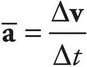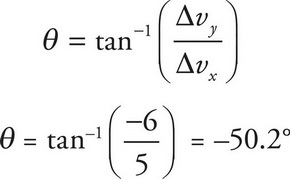# AP Physics 1 Question 374: Answer and Explanation

### Test Information

Question: 374

2. An object's initial velocity is in the second quadrant, with components v1x = -1, v1y = 4. Its final velocity is in the fourth quadrant, with components v2x = 4, v2y = -2. What is the direction of its average acceleration relative to the +x-axis?

• A. 50.2°
• B. 39.8°
• C. -39.8°
• D. -50.2°

Explanation:

D

The average acceleration is found using. Since time is a scalar that only changes the magnitude of the vector, the direction of acceleration will be defined by the change in velocity. We'll deal with each component separately.

Δvx = v2x - v1x = 4 - (-1) = 5

Δvy = v2y - v1y = -2 - (4) = -6

It may help to draw the vector Δv. The angle this vector forms with the +x-axis can be evaluated using the equation